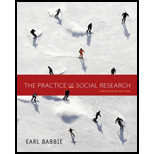Chapter 7, Problem 3RQE### The Practice of Social Research (M...

14th Edition
Earl R. Babbie
ISBN: 9781305104945

#### Solutions

Chapter
Section### The Practice of Social Research (M...

14th Edition
Earl R. Babbie
ISBN: 9781305104945
Textbook Problem

# What are the steps involved in selecting a multistage cluster sample of students taking first-year English in U.S. colleges and universities?

Summary Introduction

Introduction

Multistage cluster sampling is a way to manage complex samples. The first step is to select a sample of the clusters, then make a list of elements, and then select a sample of elements from each selected cluster.

Explanation

(This question calls for a subjective answer. This is an example of an appropriate response.)

In order to make a multistage cluster sample of students taking freshman English, it is necessary to choose a sample of clusters. In this case, the clusters might be the states where the students were born...

### Still sussing out bartleby?

Check out a sample textbook solution.

See a sample solution

#### The Solution to Your Study Problems

Bartleby provides explanations to thousands of textbook problems written by our experts, many with advanced degrees!

Get Started

## Chapter 7 Solutions